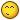# Math proof in AP or GP

## Recommended Posts

Prove that the numbers 49, 4489, 444889, 44448889,..... obtained by inserting 48 into the middle of the preceding mumbers are squares of integers.

Please give a formal mathematical proof involving Arithmetic progression or Geometric progression, not just verification.

##### Share on other sites

Any other homework you'd like done for you? Perhaps you'd like us to sit your exams for you as well?

##### Share on other sites

Any other homework you'd like done for you? Perhaps you'd like us to sit your exams for you as well?

Why would I want you to sit for my exams, the tree? You know, I am not that anxious to fail.

And for your kind notice. This is not any homework. I found this question in my Mathematics textbook for which even my teacher doesn't know the answer. I just wonder if someone could help me out.

##### Share on other sites

Well I'm stumped.

uhhh... let's see if I can at least get some kind of equation out of this.

n = distance into the progression.

let 49 be n=0.

so uh, I guess the equation would be something like

$4*10^{n+1} + 4*10^{n} + 4*10^{n-1} + ... + 4*10^{\frac{n+2}{2}} + 8*10^{\frac{n}{2}} + 8*10^{\frac{n}{2}-1} + ... 8*10^{1} + 9$

Requires n > 0 I think. Btw, I'm almost 100% sure that this is incorrect but It's probably a starting place...? Lol. I'm a bit rusty me thinks.

Edit: nevermind, my equation is rubbishMaybe someone else should take a shot.

##### Share on other sites

The digit 6 is kind of cute, at least in base 10. I kind of like this problem. So you have (7)^2 = 49, where the digits in brackets are repeated n times. This is similar to how (6)^2 = 36, which might be easier to prove.

##### Share on other sites

My apologies, you did very much give the impression that it was homework. And I'm sure you know why we shouldn't be giving homework answers. In future, maybe deliver a little more context rather than just taking a phrase out of the book.

I think CanadaAotS may have been along the right lines in trying to form a general equation to determine each term in the sequence, which would at least allow and inductive proof, if there is one.

##### Share on other sites

The way I would always do it is to find the actual thing and then use that.

This can be seen as a "displaced" geometric series, and in some way that should be able to prove everything. That is:

x_n = x_(n-1)*10 - 3. We know that for x_1 = 7, x_1^2 = 49.

x_n^2 = x_(n-1)^2*100-60*x_(n-1)+9 = _(n-1)4_(n-1)9 - 60*_(n-2)7 + 9, which should all work out.

=Uncool-

##### Share on other sites

• 4 weeks later...

i just read about this... i think.. i can solve this.. but it will be hard.. and doing it using arithmetic and geometric progression should be interesting.. and i know this is late.. so please tell me if any of u guys have already solved it haha. Also here is something interesting i saw when i approached this problem..

look.. i know this is hard to explain but look at how when we take the square root of the numbers we get like... 7, 67, 667, 6667, 66667, 666667..

square numbers 66, 666, 666, 6666, 6666 u will see that 35 is the middle number.. and for 68, 6668, 66668, 666668, u have 62 as the middle number.. its interesting when u do like 61... but there is definately a pattern. I just looked at the problem like 2 mins ago. so I haven't yet thought of a logical explanation. From what i just saw most number in the

## Create an account

Register a new account